# Covert C++ code into MIPS. -If x has a value if 2, print "bbb" if x...

Covert C++ code into MIPS.

-If x has a value if 2, print "bbb" if x has a value of 3, print "ccc" if x has a value of 4, print "ddd" if x has a value other than 2, 3, or 4 print "eee".

result = "";
switch (x)
{
case 2: result = result + "bbb"; break;
case 3: result = result + "ccc"; break;
case 4: result = result + "ddd"; break;
default: result = result + "eee";
}
cout << "3.\t" << result << endl;

This C++ code in MIPS? Need help.

please find the required C++ program:

//==========================================

#include <iostream>

#include<string.h>

using namespace std;

int main() {

string result = "";

int x;

switch (x)

{

case 2: result = result + "bbb"; break;

case 3: result = result + "ccc"; break;

case 4: result = result + "ddd"; break;

default: result = result + "eee";

}

cout << "3.\t" << result << endl;

return 1;

}

//==========================================

corresponding MIPS program:

//===========================================

.LC0:

.ascii "\000"

.LC1:

.ascii "bbb\000"

.LC2:

.ascii "ccc\000"

.LC3:

.ascii "ddd\000"

.LC4:

.ascii "eee\000"

.LC5:

.ascii "3.\011\000"

main:

daddiu $sp,$sp,-208

sd $31,200($sp)

sd $fp,192($sp)

sd $28,184($sp)

sd $16,176($sp)

move $fp,$sp

lui $28,%hi(%neg(%gp_rel(main))) daddu$28,$28,$25

daddiu $28,$28,%lo(%neg(%gp_rel(main)))

daddiu $2,$fp,136

move $4,$2

ld $2,%call16(_ZNSaIcEC1Ev)($28)

move $25,$2

1: jalr $25 nop daddiu$2,$fp,136 daddiu$3,$fp,144 move$6,$2 ld$2,%got_page(.LC0)($28) daddiu$5,$2,%got_ofst(.LC0) move$4,$3 ld$2,%call16(_ZNSt7__cxx1112basic_stringIcSt11char_traitsIcESaIcEEC1EPKcRKS3_)($28) move$25,$2 1: jalr$25

nop

daddiu $2,$fp,136

move $4,$2

ld $2,%call16(_ZNSaIcED1Ev)($28)

move $25,$2

1: jalr $25 nop lw$2,0($fp) li$3,3 # 0x3

beq $2,$3,.L3

nop

li $3,4 # 0x4 beq$2,$3,.L4 nop li$3,2 # 0x2

bne $2,$3,.L20

nop

daddiu $3,$fp,104

daddiu $4,$fp,144

ld $2,%got_page(.LC1)($28)

daddiu $6,$2,%got_ofst(.LC1)

move $5,$4

move $4,$3

ld $2,%got_disp(std::__cxx11::basic_string<char, std::char_traits<char>, std::allocator<char> > std::operator+<char, std::char_traits<char>, std::allocator<char> >(std::__cxx11::basic_string<char, std::char_traits<char>, std::allocator<char> > const&, char const*))($28)

move $25,$2

1: jalr $25 nop daddiu$3,$fp,104 daddiu$2,$fp,144 move$5,$3 move$4,$2 ld$2,%call16(_ZNSt7__cxx1112basic_stringIcSt11char_traitsIcESaIcEEaSERKS4_)($28) move$25,$2 1: jalr$25

nop

daddiu $2,$fp,104

move $4,$2

ld $2,%call16(_ZNSt7__cxx1112basic_stringIcSt11char_traitsIcESaIcEED1Ev)($28)

move $25,$2

1: jalr $25 nop b .L6 nop .L3: daddiu$3,$fp,72 daddiu$4,$fp,144 ld$2,%got_page(.LC2)($28) daddiu$6,$2,%got_ofst(.LC2) move$5,$4 move$4,$3 ld$2,%got_disp(std::__cxx11::basic_string<char, std::char_traits<char>, std::allocator<char> > std::operator+<char, std::char_traits<char>, std::allocator<char> >(std::__cxx11::basic_string<char, std::char_traits<char>, std::allocator<char> > const&, char const*))($28) move$25,$2 1: jalr$25

nop

daddiu $3,$fp,72

daddiu $2,$fp,144

move $5,$3

move $4,$2

ld $2,%call16(_ZNSt7__cxx1112basic_stringIcSt11char_traitsIcESaIcEEaSERKS4_)($28)

move $25,$2

1: jalr $25 nop daddiu$2,$fp,72 move$4,$2 ld$2,%call16(_ZNSt7__cxx1112basic_stringIcSt11char_traitsIcESaIcEED1Ev)($28) move$25,$2 1: jalr$25

nop

b .L6

nop

.L4:

daddiu $3,$fp,40

daddiu $4,$fp,144

ld $2,%got_page(.LC3)($28)

daddiu $6,$2,%got_ofst(.LC3)

move $5,$4

move $4,$3

ld $2,%got_disp(std::__cxx11::basic_string<char, std::char_traits<char>, std::allocator<char> > std::operator+<char, std::char_traits<char>, std::allocator<char> >(std::__cxx11::basic_string<char, std::char_traits<char>, std::allocator<char> > const&, char const*))($28)

move $25,$2

1: jalr $25 nop daddiu$3,$fp,40 daddiu$2,$fp,144 move$5,$3 move$4,$2 ld$2,%call16(_ZNSt7__cxx1112basic_stringIcSt11char_traitsIcESaIcEEaSERKS4_)($28) move$25,$2 1: jalr$25

nop

daddiu $2,$fp,40

move $4,$2

ld $2,%call16(_ZNSt7__cxx1112basic_stringIcSt11char_traitsIcESaIcEED1Ev)($28)

move $25,$2

1: jalr $25 nop b .L6 nop .L20: daddiu$3,$fp,8 daddiu$4,$fp,144 ld$2,%got_page(.LC4)($28) daddiu$6,$2,%got_ofst(.LC4) move$5,$4 move$4,$3 ld$2,%got_disp(std::__cxx11::basic_string<char, std::char_traits<char>, std::allocator<char> > std::operator+<char, std::char_traits<char>, std::allocator<char> >(std::__cxx11::basic_string<char, std::char_traits<char>, std::allocator<char> > const&, char const*))($28) move$25,$2 1: jalr$25

nop

daddiu $3,$fp,8

daddiu $2,$fp,144

move $5,$3

move $4,$2

ld $2,%call16(_ZNSt7__cxx1112basic_stringIcSt11char_traitsIcESaIcEEaSERKS4_)($28)

move $25,$2

1: jalr $25 nop daddiu$2,$fp,8 move$4,$2 ld$2,%call16(_ZNSt7__cxx1112basic_stringIcSt11char_traitsIcESaIcEED1Ev)($28) move$25,$2 1: jalr$25

nop

.L6:

ld $2,%got_page(.LC5)($28)

daddiu $5,$2,%got_ofst(.LC5)

ld $4,%got_disp(_ZSt4cout)($28)

ld $2,%call16(_ZStlsISt11char_traitsIcEERSt13basic_ostreamIcT_ES5_PKc)($28)

move $25,$2

1: jalr $25 nop move$3,$2 daddiu$2,$fp,144 move$5,$2 move$4,$3 ld$2,%call16(_ZStlsIcSt11char_traitsIcESaIcEERSt13basic_ostreamIT_T0_ES7_RKNSt7__cxx1112basic_stringIS4_S5_T1_EE)($28) move$25,$2 1: jalr$25

nop

ld $5,%got_disp(_ZSt4endlIcSt11char_traitsIcEERSt13basic_ostreamIT_T0_ES6_)($28)

move $4,$2

ld $2,%call16(_ZNSolsEPFRSoS_E)($28)

move $25,$2

1: jalr $25 nop li$16,1 # 0x1

daddiu $2,$fp,144

move $4,$2

ld $2,%call16(_ZNSt7__cxx1112basic_stringIcSt11char_traitsIcESaIcEED1Ev)($28)

move $25,$2

1: jalr $25 nop move$2,$16 b .L21 nop move$16,$4 daddiu$2,$fp,136 move$4,$2 ld$2,%call16(_ZNSaIcED1Ev)($28) move$25,$2 1: jalr$25

nop

move $2,$16

move $4,$2

ld $2,%call16(_Unwind_Resume)($28)

move $25,$2

1: jalr $25 nop move$16,$4 daddiu$2,$fp,104 move$4,$2 ld$2,%call16(_ZNSt7__cxx1112basic_stringIcSt11char_traitsIcESaIcEED1Ev)($28) move$25,$2 1: jalr$25

nop

b .L10

nop

move $16,$4

daddiu $2,$fp,72

move $4,$2

ld $2,%call16(_ZNSt7__cxx1112basic_stringIcSt11char_traitsIcESaIcEED1Ev)($28)

move $25,$2

1: jalr $25 nop b .L10 nop move$16,$4 daddiu$2,$fp,40 move$4,$2 ld$2,%call16(_ZNSt7__cxx1112basic_stringIcSt11char_traitsIcESaIcEED1Ev)($28) move$25,$2 1: jalr$25

nop

b .L10

nop

move $16,$4

daddiu $2,$fp,8

move $4,$2

ld $2,%call16(_ZNSt7__cxx1112basic_stringIcSt11char_traitsIcESaIcEED1Ev)($28)

move $25,$2

1: jalr $25 nop b .L10 nop move$16,$4 .L10: daddiu$2,$fp,144 move$4,$2 ld$2,%call16(_ZNSt7__cxx1112basic_stringIcSt11char_traitsIcESaIcEED1Ev)($28) move$25,$2 1: jalr$25

nop

move $2,$16

move $4,$2

ld $2,%call16(_Unwind_Resume)($28)

move $25,$2

1: jalr $25 nop .L21: move$sp,$fp ld$31,200($sp) ld$fp,192($sp) ld$28,184($sp) ld$16,176($sp) daddiu$sp,$sp,208 j$31

nop

std::__cxx11::basic_string<char, std::char_traits<char>, std::allocator<char> > std::operator+<char, std::char_traits<char>, std::allocator<char> >(std::__cxx11::basic_string<char, std::char_traits<char>, std::allocator<char> > const&, char const*):

daddiu $sp,$sp,-64

sd $31,56($sp)

sd $fp,48($sp)

sd $28,40($sp)

sd $16,32($sp)

move $fp,$sp

lui $28,%hi(%neg(%gp_rel(std::__cxx11::basic_string<char, std::char_traits<char>, std::allocator<char> > std::operator+<char, std::char_traits<char>, std::allocator<char> >(std::__cxx11::basic_string<char, std::char_traits<char>, std::allocator<char> > const&, char const*)))) daddu$28,$28,$25

daddiu $28,$28,%lo(%neg(%gp_rel(std::__cxx11::basic_string<char, std::char_traits<char>, std::allocator<char> > std::operator+<char, std::char_traits<char>, std::allocator<char> >(std::__cxx11::basic_string<char, std::char_traits<char>, std::allocator<char> > const&, char const*))))

sd $4,0($fp)

sd $5,8($fp)

sd $6,16($fp)

ld $5,8($fp)

ld $4,0($fp)

ld $2,%call16(_ZNSt7__cxx1112basic_stringIcSt11char_traitsIcESaIcEEC1ERKS4_)($28)

move $25,$2

1: jalr $25 nop ld$5,16($fp) ld$4,0($fp) ld$2,%call16(_ZNSt7__cxx1112basic_stringIcSt11char_traitsIcESaIcEE6appendEPKc)($28) move$25,$2 1: jalr$25

nop

b .L26

nop

move $16,$4

ld $4,0($fp)

ld $2,%call16(_ZNSt7__cxx1112basic_stringIcSt11char_traitsIcESaIcEED1Ev)($28)

move $25,$2

1: jalr $25 nop move$2,$16 move$4,$2 ld$2,%call16(_Unwind_Resume)($28) move$25,$2 1: jalr$25

nop

.L26:

ld $2,0($fp)

move $sp,$fp

ld $31,56($sp)

ld $fp,48($sp)

ld $28,40($sp)

ld $16,32($sp)

daddiu $sp,$sp,64

j $31 nop __static_initialization_and_destruction_0(int, int): daddiu$sp,$sp,-48 sd$31,40($sp) sd$fp,32($sp) sd$28,24($sp) move$fp,$sp lui$28,%hi(%neg(%gp_rel(__static_initialization_and_destruction_0(int, int))))

daddu $28,$28,$25 daddiu$28,$28,%lo(%neg(%gp_rel(__static_initialization_and_destruction_0(int, int)))) move$3,$4 move$2,$5 sll$3,$3,0 sw$3,0($fp) sll$2,$2,0 sw$2,4($fp) lw$3,0($fp) li$2,1 # 0x1

bne $3,$2,.L29

nop

lw $3,4($fp)

li $2,65535 # 0xffff bne$3,$2,.L29 nop ld$2,%got_page(_ZStL8__ioinit)($28) daddiu$4,$2,%got_ofst(_ZStL8__ioinit) ld$2,%call16(_ZNSt8ios_base4InitC1Ev)($28) move$25,$2 1: jalr$25

nop

ld $6,%got_disp(__dso_handle)($28)

ld $2,%got_page(_ZStL8__ioinit)($28)

daddiu $5,$2,%got_ofst(_ZStL8__ioinit)

ld $4,%got_disp(_ZNSt8ios_base4InitD1Ev)($28)

ld $2,%call16(__cxa_atexit)($28)

move $25,$2

1: jalr $25 nop .L29: nop move$sp,$fp ld$31,40($sp) ld$fp,32($sp) ld$28,24($sp) daddiu$sp,$sp,48 j$31

nop

_GLOBAL__sub_I_main:

daddiu $sp,$sp,-32

sd $31,24($sp)

sd $fp,16($sp)

sd $28,8($sp)

move $fp,$sp

lui $28,%hi(%neg(%gp_rel(_GLOBAL__sub_I_main))) daddu$28,$28,$25

daddiu $28,$28,%lo(%neg(%gp_rel(_GLOBAL__sub_I_main)))

li $5,65535 # 0xffff li$4,1 # 0x1

ld $2,%got_page(__static_initialization_and_destruction_0(int, int))($28)

daddiu $2,$2,%got_ofst(__static_initialization_and_destruction_0(int, int))

move $25,$2

1: jalr $25 nop move$sp,$fp ld$31,24($sp) ld$fp,16($sp) ld$28,8($sp) daddiu$sp,$sp,32 j$31

nop

//===========================================

##### Add Answer to: Covert C++ code into MIPS. -If x has a value if 2, print "bbb" if x...
Similar Homework Help Questions
• ### Convert the below code into if else selection: #include <iostream> using namespace std; int main() {...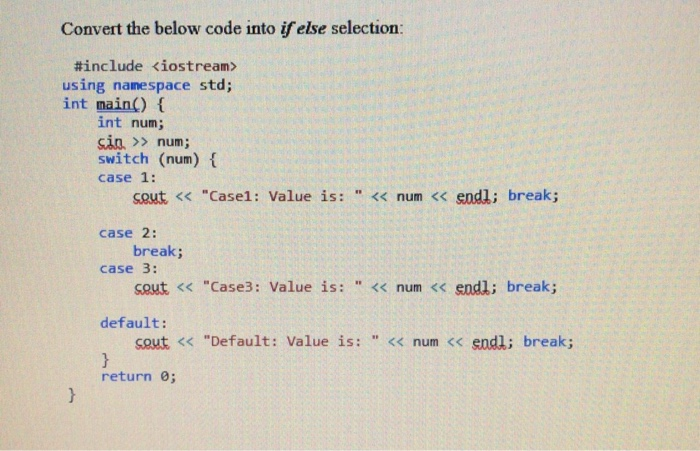Convert the below code into if else selection: #include <iostream> using namespace std; int main() { int num; sin. >> num; switch (num) { case 1: cout << "Casel: Value is: << num << endl; break; case 2: break; case 3: cout << "Case3: Value is: " << num << endl; break; default: cout << "Default: Value is: << num << endl; break; } return; }

• ### Question 5: Consider the following C++ program. switch(x) case 1: if (x > 1) case 2:...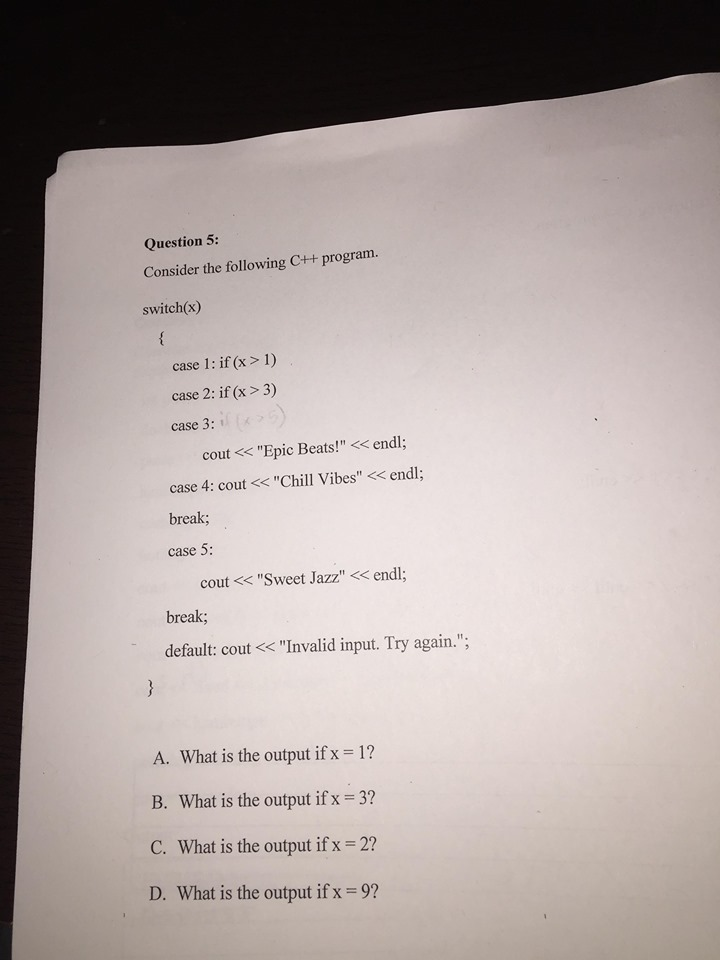Question 5: Consider the following C++ program. switch(x) case 1: if (x > 1) case 2: if (x > 3) case 3: cout<<"Epic Beats!" << endl; case 4: cout << "Chill Vibes" << endl; break; case 5: cout << "Sweet Jazz" << endl; break; default: cout << "Invalid input. Try again."; A. What is the output if x = 1? B. What is the output if x = 3? C. What is the output if x = 2? D. What...

• ### Convert the following code to assembly MIPS void checki() { int i = 0; while(i <...

Convert the following code to assembly MIPS void checki() { int i = 0; while(i < 10) { switch(i) { case 1: i += 5; break; case 2: i --; break; case 3: i += 2; break; default: i++; } } } void main() { checki(); }

• ### C++ Object Oriented assignment Can you please check the program written below if it has appropriately...

C++ Object Oriented assignment Can you please check the program written below if it has appropriately fulfilled the instructions provided below. Please do the necessary change that this program may need. I am expecting to get a full credit for this assignment so put your effort to correct and help the program have the most efficient algorithm within the scope of the instruction given. INSTRUCTIONS Create a fraction class and add your Name to the name fraction and use this...

• ### C++ Banks offer various types of accounts, such as savings, checking, certificate of deposits, and money...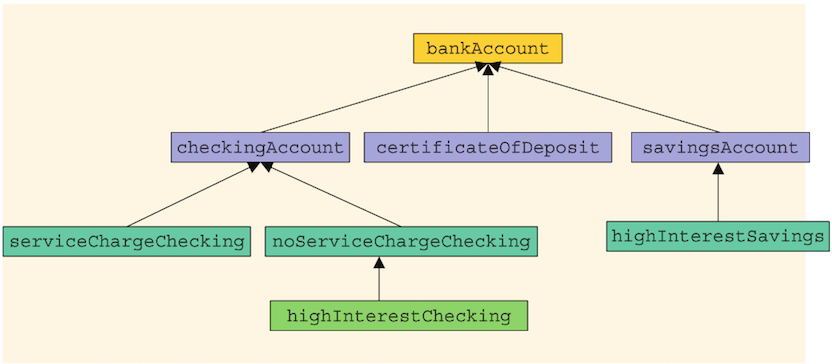C++ Banks offer various types of accounts, such as savings, checking, certificate of deposits, and money market, to attract customers as well as meet their specific needs. Two of the most commonly used accounts are savings and checking. Each of these accounts has various options. For example, you may have a savings account that requires no minimum balance but has a lower interest rate. Similarly, you may have a checking account that limits the number of checks you may write....

• ### 7 - What does the code print to the screen? float x(float) 7/3; printf ( "...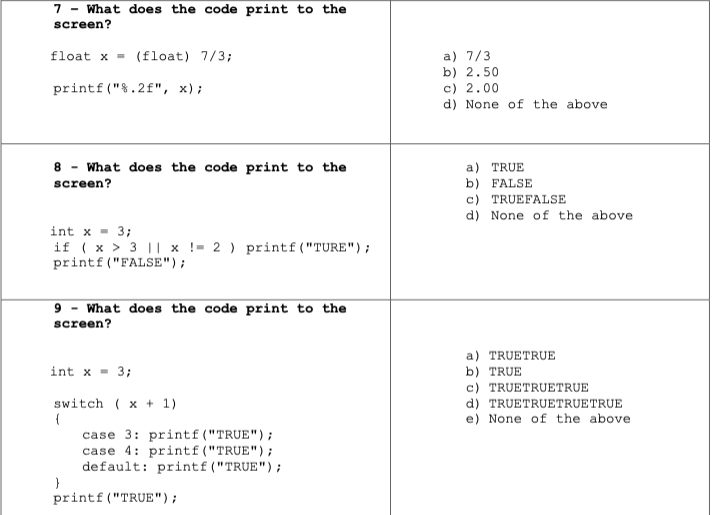7 - What does the code print to the screen? float x(float) 7/3; printf ( " % .2 f", x); a) 7/3 b) 2.50 c) 2.00 d) None of the above a) TRUE b) FALSE c) TRUEFALSE d) None of the above 8 - What does the code print to the screen? int x3; if (x >31x!-2 printf ("TURE") printf ("FALSE"); 9What does the code print to the screen? a) TRUETRUE b) TRUE c) TRUETRUETRUE d) TRUETRUETRUETRUE e) None of...

• ### C++ Programming

7. What is the output of the following code fragment?int x = 100;int y = 200;if (x > 100 &&y <= 200)cout<< x <<""<< y <<""<< x + y <<endl;elsecout<< x <<""<< y <<""<< 2 * x - y <<endl;8. Given a char variable called gender, write C++ statements to output"Male" if gender contains upper or lower case M, "Female" if it contains upper or lower case F,or "Invalid Gender" if it contains anything else. Use if / else if...

• ### In C++ please! *Do not use any other library functions(like strlen) than the one posted(<cstddef>) in the code below!* /** CS 150 C-Strings Follow the instructions on your handout to complete t...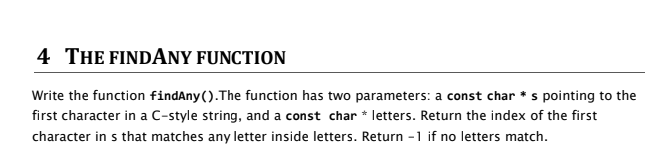In C++ please! *Do not use any other library functions(like strlen) than the one posted(<cstddef>) in the code below!* /** CS 150 C-Strings Follow the instructions on your handout to complete the requested function. You may not use ANY library functions or include any headers, except for <cstddef> for size_t. */ #include <cstddef> // size_t for sizes and indexes ///////////////// WRITE YOUR FUNCTION BELOW THIS LINE /////////////////////// ///////////////// WRITE YOUR FUNCTION ABOVE THIS LINE /////////////////////// // These are OK after...

• ### I need help solving this assignment, you are given a full piece of code that does not run correctly. Some of the bugs ca...

I need help solving this assignment, you are given a full piece of code that does not run correctly. Some of the bugs can be found at compile time, while others are found at run time. Access the UserMenu.cpp code file (in the zip file in Start Here) and use debugging practices and the debugger in Visual Studio to find each bug. Fix the bug and write a comment in the code giving a description of how you found the...

• ### Transfer C code of selection sort to MIPS code and print the sorted array/results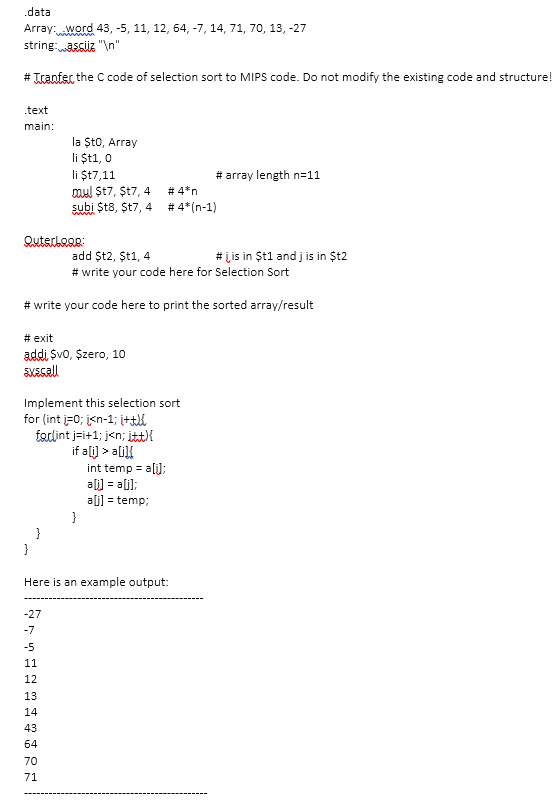Transfer C code of selection sort to MIPS code and print the sorted array/results data Array: word 43, -5, 11, 12, 64, -7, 14, 71, 70, 13, -27 string: asciz"In" # Trantec the C code of selection sort to MIPS code. Do not modify the existing code and structure! text main la ŞtO, Array li $t1, 0 li$t7,11 mul $17,$17, 4 subi $t8,$t7, 4 # array length n-11 # 4*n #4*(n-1) # lis in \$t1 and j is...

Free Homework App